# Week 2 — Feature Engineering

## Introduction to Preprocessing

Feature engineering can be difficult and time consuming, but also very important to success.

### Squeezing the most out of data

• Making data useful before training a model
• Representing data in forms that help models learn
• Increasing predictive quality
• Reducing dimensionality with feature engineering
• Feature Engineering within the model is limited to batch computations

### Art of feature engineering

Increases the model ability to learn while simultaneously reducing (if possible) the compute resources it requires.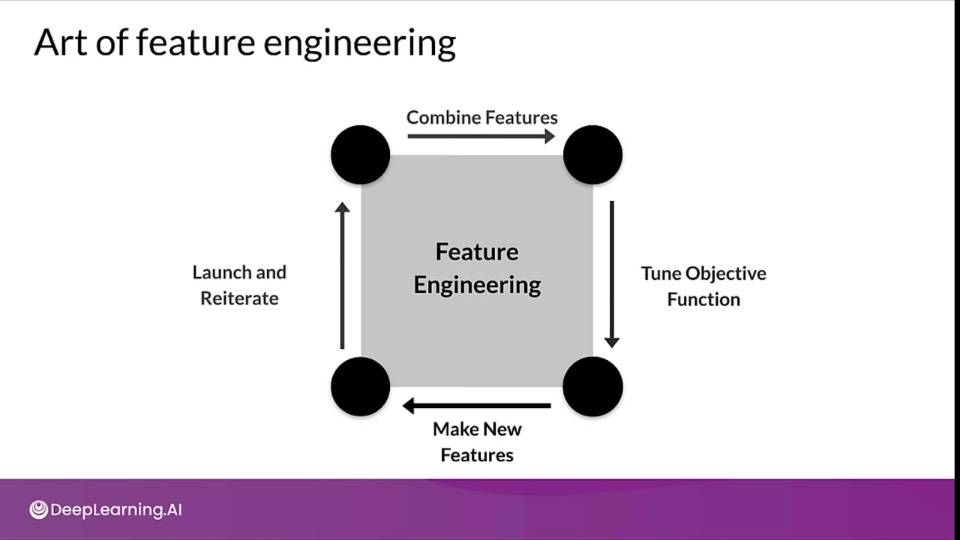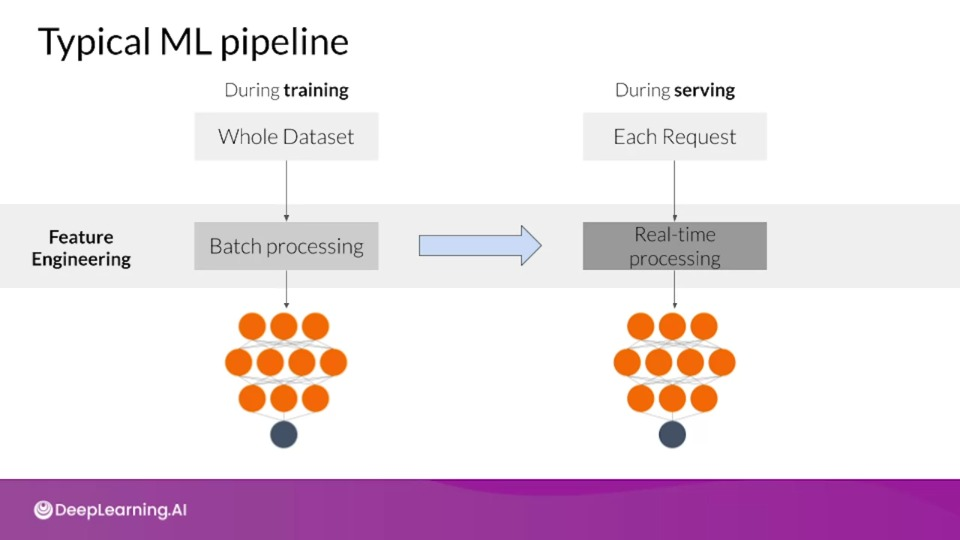During serving we typically process each request individually, so it becomes important that we include global properties of our features, such as the $\sigma$ (standard deviation)

## Preprocessing Operations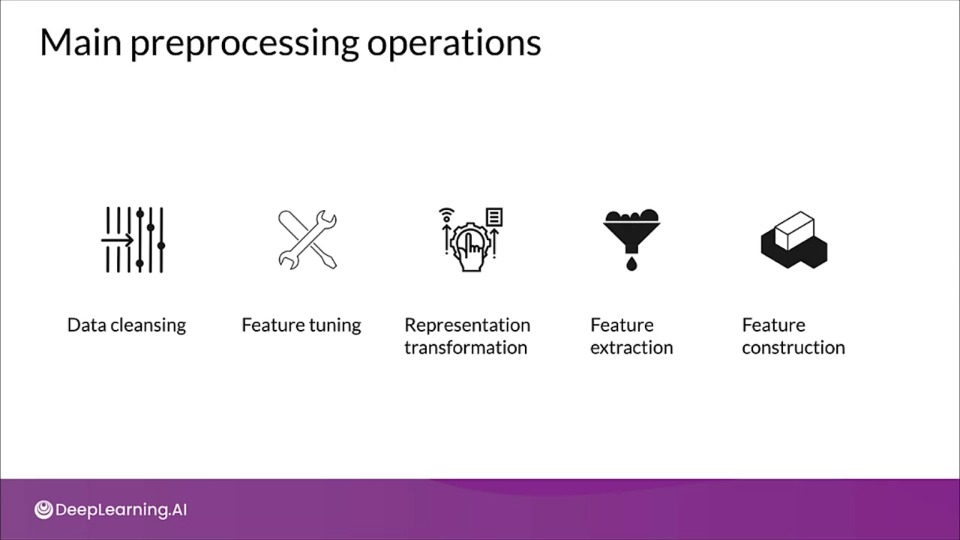Data clearning to remove erroneous data.

Feature tuning like normalizing, scaling.

Representation transformation for better predictive signals

Feature Extraction / dimensionality reduction for more data representation.

Feature construction to create new features.

### Mapping categorical values

Categorical values can be one-hot encoded if two nearby values are not more similar than two distant values, otherwise ordinal encoded.

### Empirical knowledge of data will guide you further

Text: stemming, lemmatization, TF-IDF, n-grams, embedding lookup

Images - clipping, resizing, cropping, blur, canny filters, soble filters, photometric distortions

### Key points

• Data preprocessing: transforms raw data into a clean and training-ready dataset
• Feature engineering maps:
• Raw data into feature vectors
• Integer values to floating-point values
• Normalizes numerical values
• String and categorical values to vectors of numeric values
• Data from one space into different space

## Feature Engineering Techniques

### Scaling

• Converts values from their natural range into a prescribed range
• e.g., grayscale image pixel intensity scale is $[0, 255]$ usually rescaled to $[-1, 1]$

$$x_\text{scaled} = \frac{(b-a)(x - x_{\min})}{x_{\max} - x_{\min}} + a \tag{x \isin [a, b]}$$

Normalization:

$$x_\text{scaled} = \frac{x - x_{\min}}{x_{\max} - x_{\min}} \tag{x \isin [0, 1]}$$

• Benefits
• Helps NN converge faster
• Do away with NaN errors during training
• For each feature, the model learn the right weights.

### Standardization

• Z-score relates the number of standard deviations away from the mean

$$x_\text{std} = \frac{x - \mu}{\sigma}$$

### Bucketizing/ Binning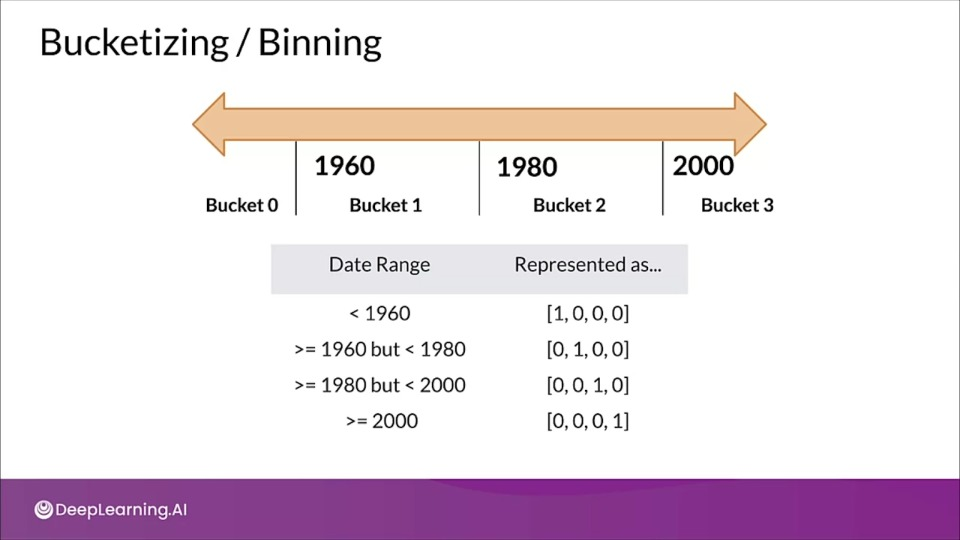### Other techniques

Dimensionality reduction in embeddings

• Principle component analysis (PCA)
• t-Distribute stochastic neighbor embedding (t-SNE)
• uniform manifold approximation and projection (UMAP)

### TensorFlow embedding projector

• Intuitive explanation of high-dimensional data
• Visualize & analyze

## Feature Crosses

• Combine multiple features together into a new feature
• Encodes nonlinearity in the feature space, or encodes the same information in fewer features
• $[A \times B]$ : multiplying the values of two features
• $[A\times B\times C \times D \times E ]$: multiplying the values of 5 features
• $[\text{Day of week, hour}] \rightarrow [\text{Hour of week}]$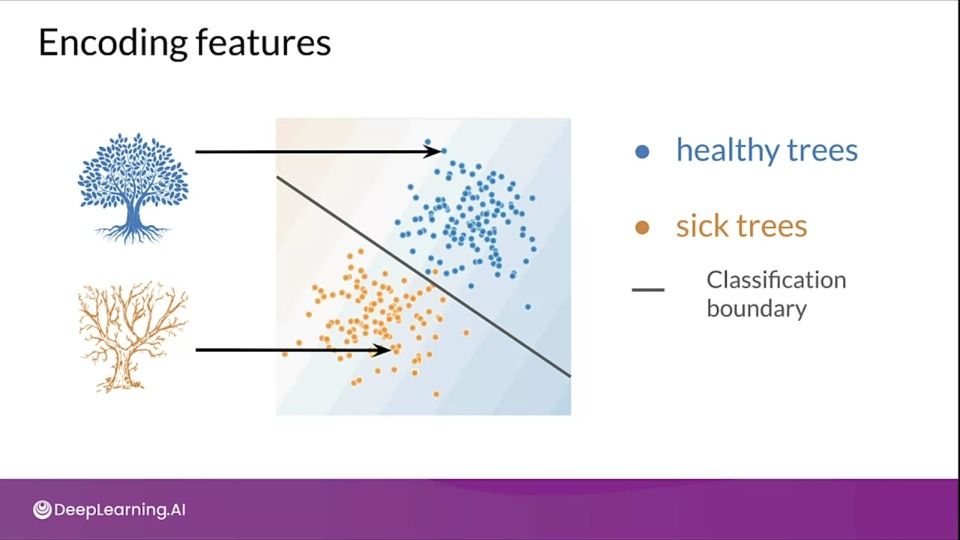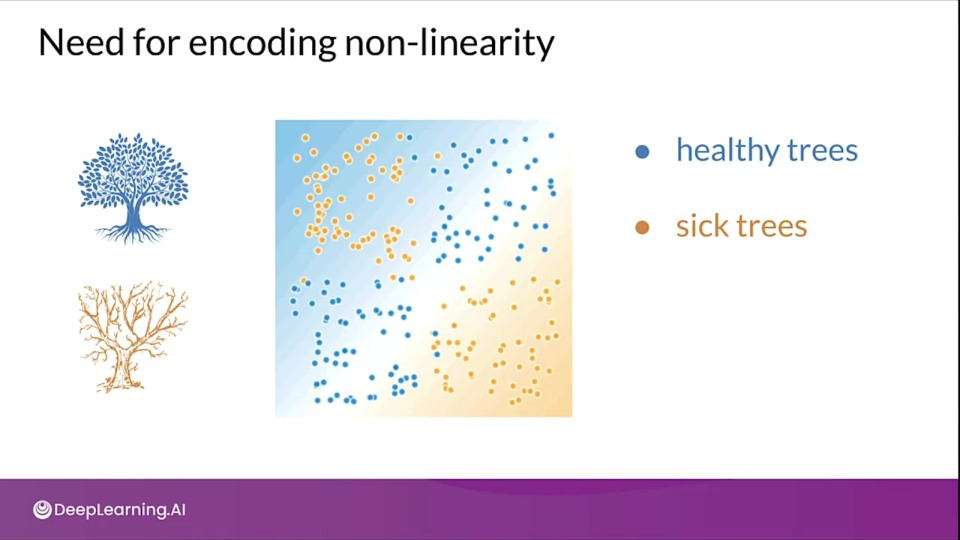### Key points

• Feature crossing: synthetic feature encoding nonlinearity in feature space
• Feature coding: Transforming categorical to a continuous variable.

## Feature Transformation at Scale — Preprocessing Data at Scale

To do feature transformation at scale we need ML pipeline to deploy our model with consistent and reproducible results.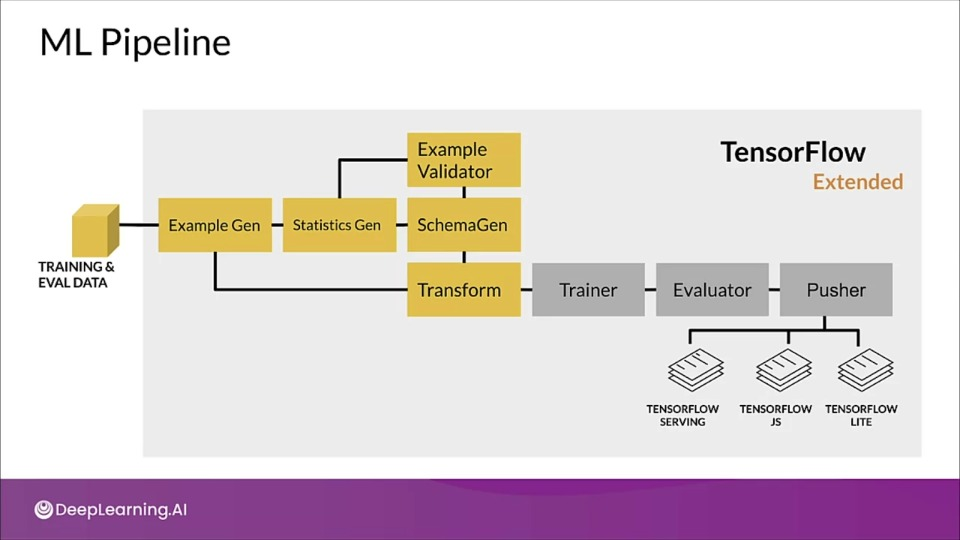### Preprocessing at scale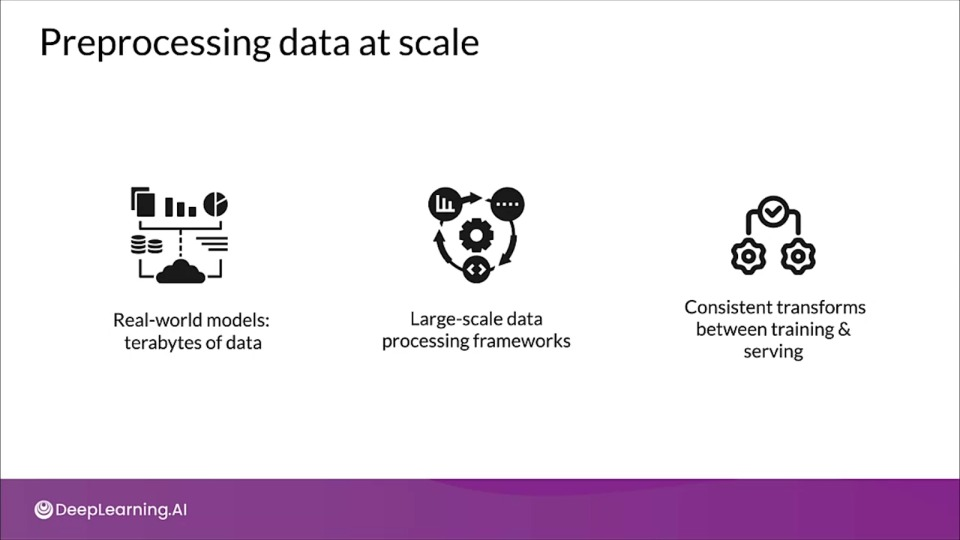### Inconsistencies in feature engineering

• Training & serving code paths are different
• Diverse deployment scenarios
• Mobile (TensorFlow Lite)
• Server (TensorFlow Serving)
• Web (TensorFlow JS)
• Risks of introducing training-serving skews
• Skews will lower the performance of your serving model

### Preprocessing granularity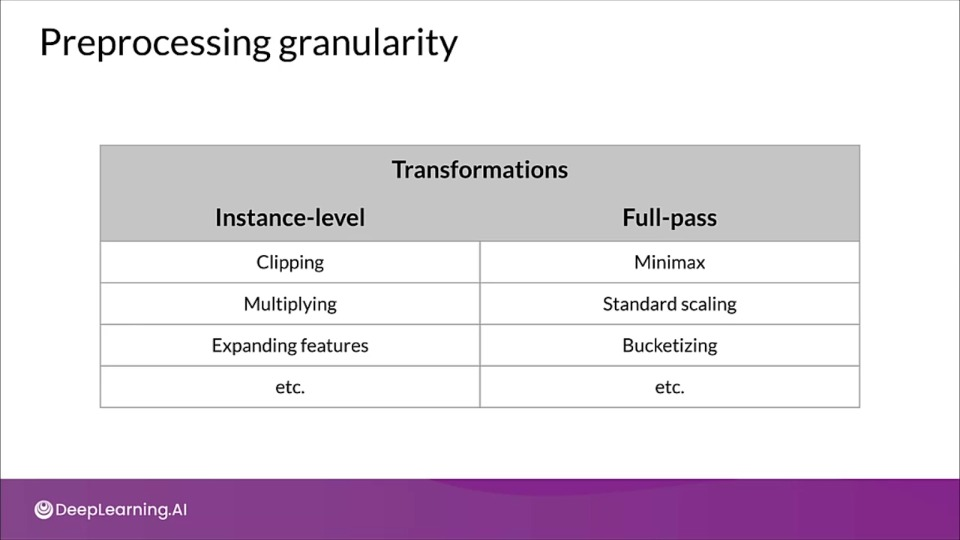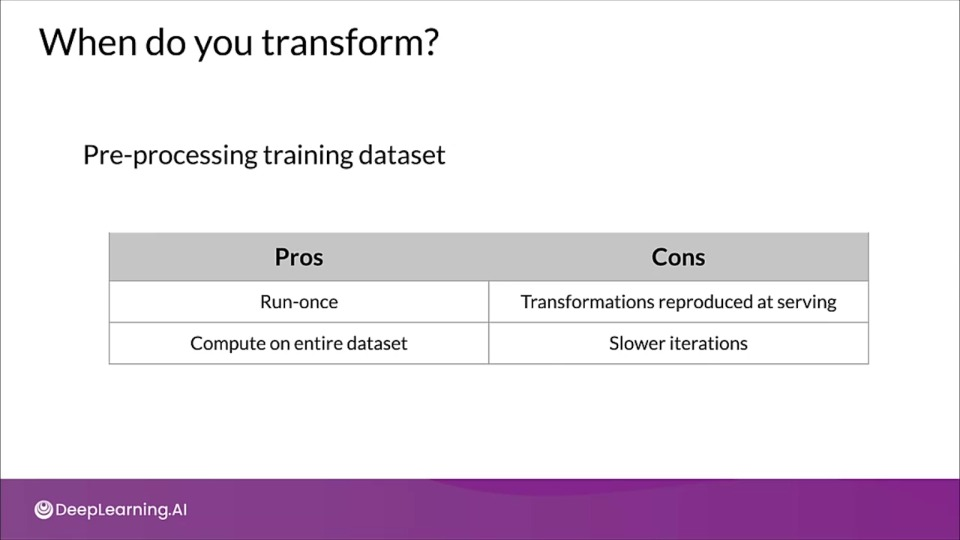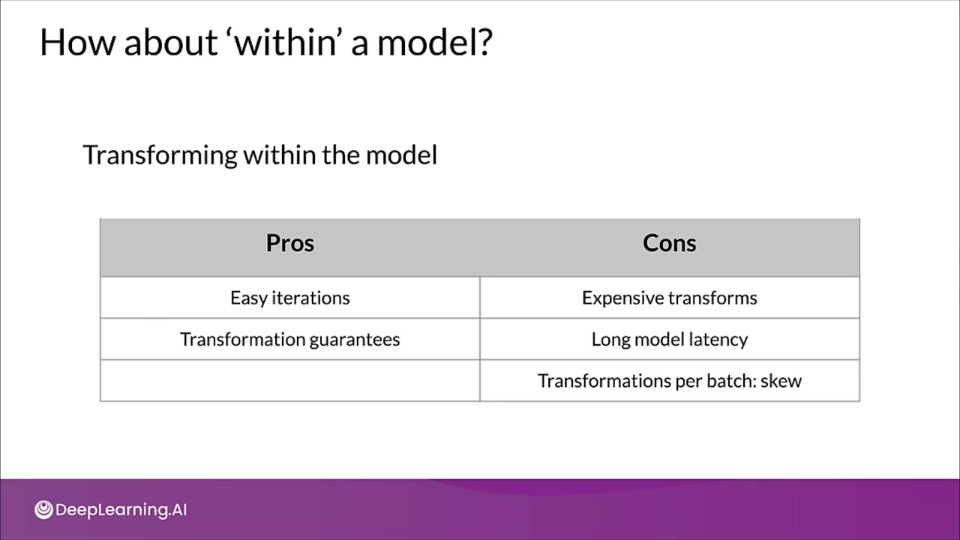### Applying Transformation per batch

• For example, normalizing features by their average
• Access to a single batch of data, not the full dataset
• Ways to normalize per batch
• Normalize by average within a batch
• Precompute average and reuse it during normalization

### Optimizing instance-level transformations

• Indirectly affect training efficeincy
• Typically accelerators sit idle while the CPUs transform
• Solution:
• Prefetching transforms for better accelerator efficiency

### Summarizing the challenges

• Balancing predictive performance
• Full-pass transformation on training data
• Optimizing instance-level transformation for better training efficiency (GPUs, TPUs,...)

### Key points

• Inconsistent data affects the accuracy of the results
• Need for scaled data processing frameworks to process large datasets in an efficient and distribute manner

## TensorFlow Transform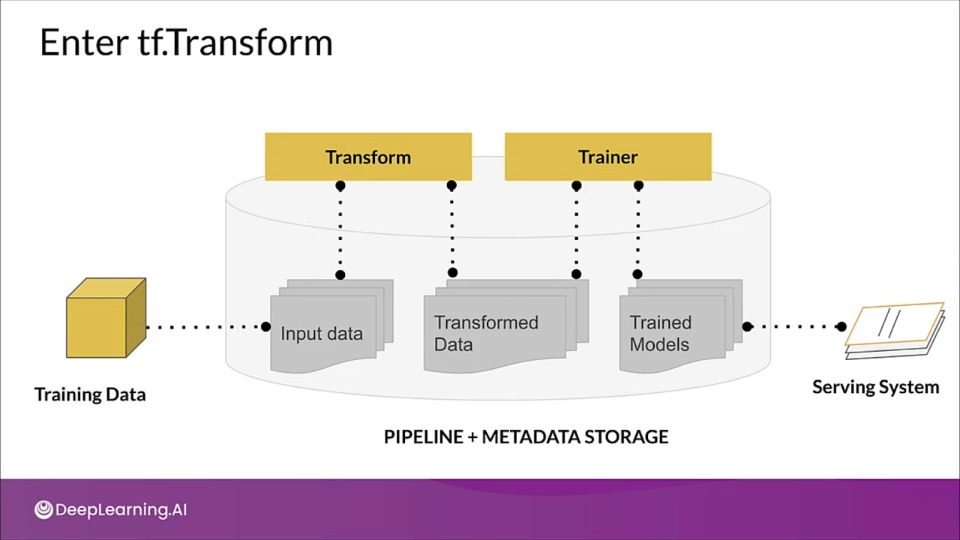Example Gen: Generates Examples from the training & evaluation data

Statistics Gen: Generates Statistics

Schema Gen: Generates schema after ingesting statistics. This schema is then fed to:

• Example validator: Takes schema and statistics and look for problems/anomalies in data
• Transform: takes schema and dataset and do feature engineering

Trainer: Trains the model

Evaluator: Evaluates the result

Pusher: Pushes to wherever we want to serve our model.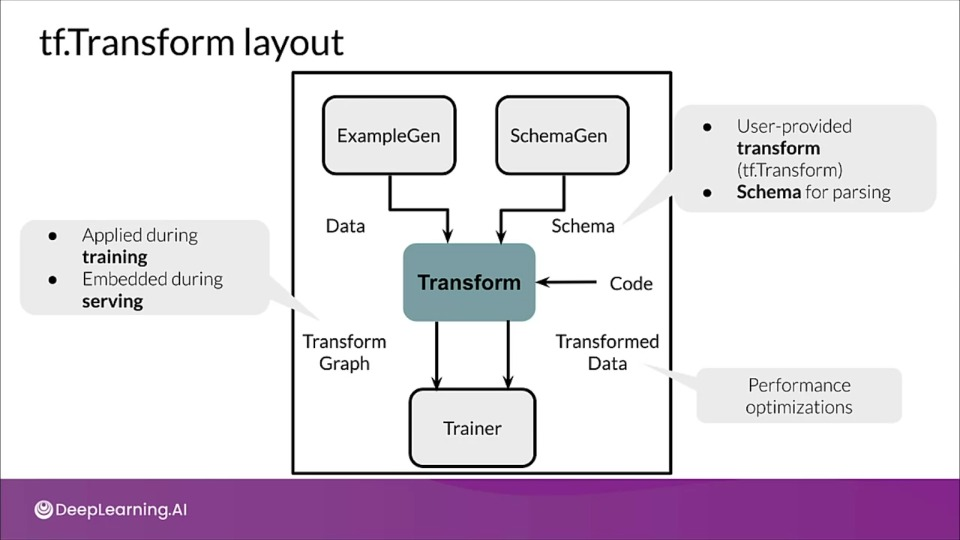### tf.Transform: Going Deeper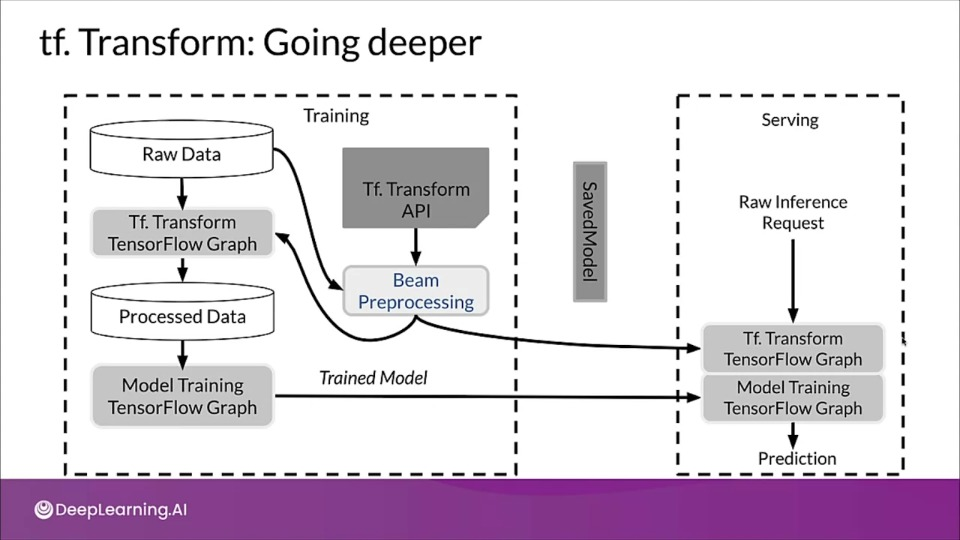### tf.Transform Analyzers

Analyzers make a full pass over the dataset in order to collect constants that is required to do feature engineering. It also express the operations that we are going to do.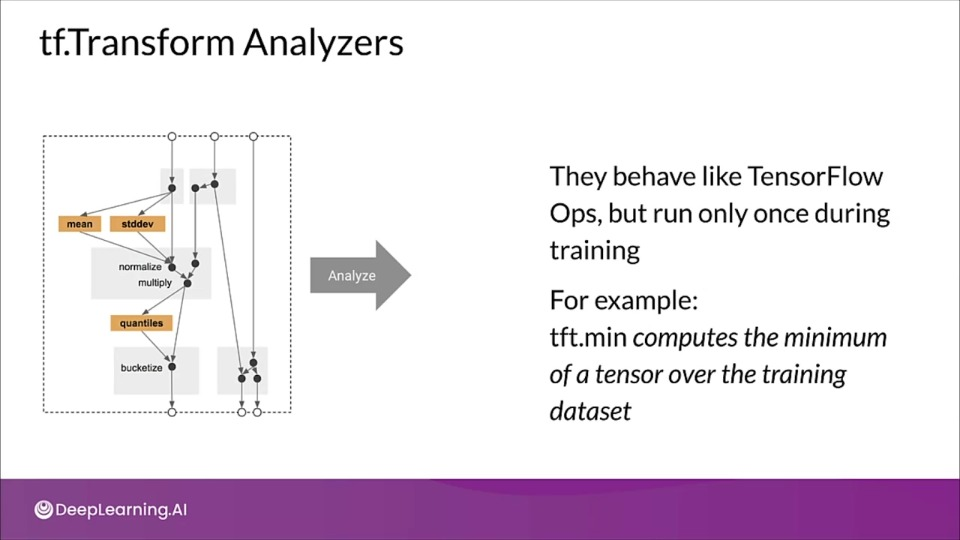### How Transform applies feature transformations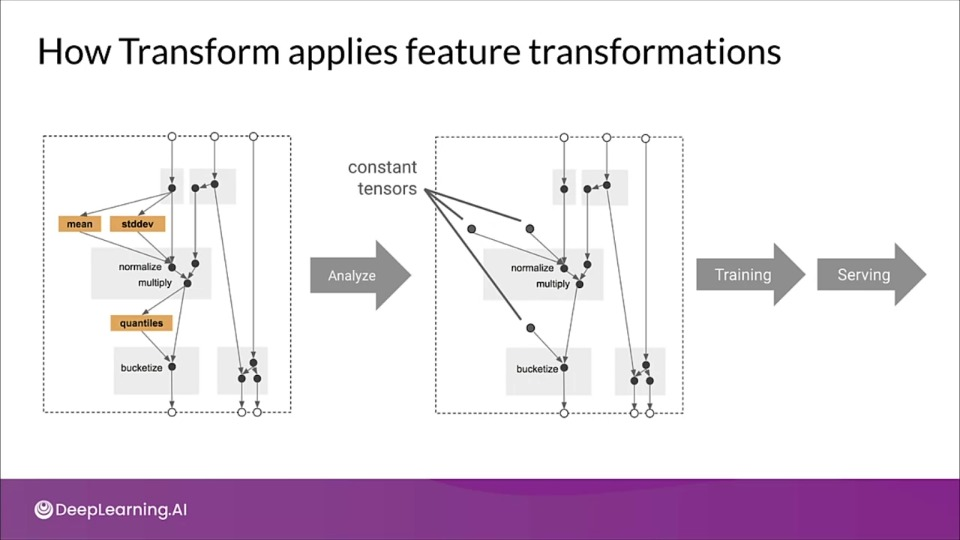### Benefits of using tf.Transform

• Emitted tf.Graph holds all necessary constants and transformations
• Focus on data preprocessing only at training time
• Works in-line during both training and serving
• No need for preprocessing code at serving time
• Consistently applied transformations irrespective of deployment platform

### Analyzers framework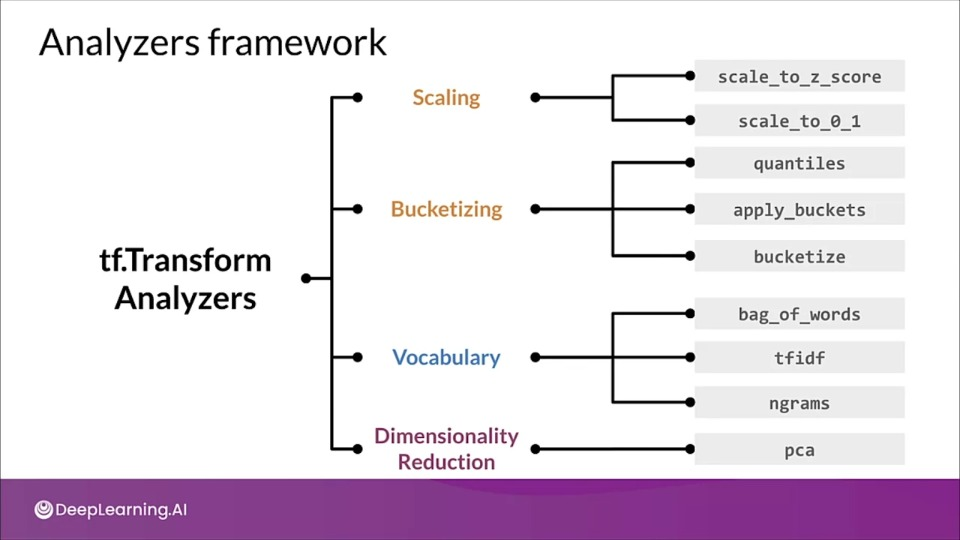### tf.Transform preprocessing_fn

def preprocessing_fn(inputs):
...
for key in DENSE_FLOAT_FEATURE_KEYS:
outputs[key] = tft.scale_to_z_score(inputs[key])

for key in VOCAB_FEATURE_KEYS:
outputs[key] = tft.vocabulary(inputs[key], vocab_filename=key)

for jey in BUCKET_FEATURE_KEYS:
outputs[key] = tft.bucketize(inputs[key], FEATURE_BUCKET_COUNT)


### Commonly Used Imports

import tensorflow as tf
import apache_beam as beam
import apache_beam.io.iobase

import tensorflow_transform as tft
import tensorflow_transform.beam as tft_beam


## Hello World with tf.Transform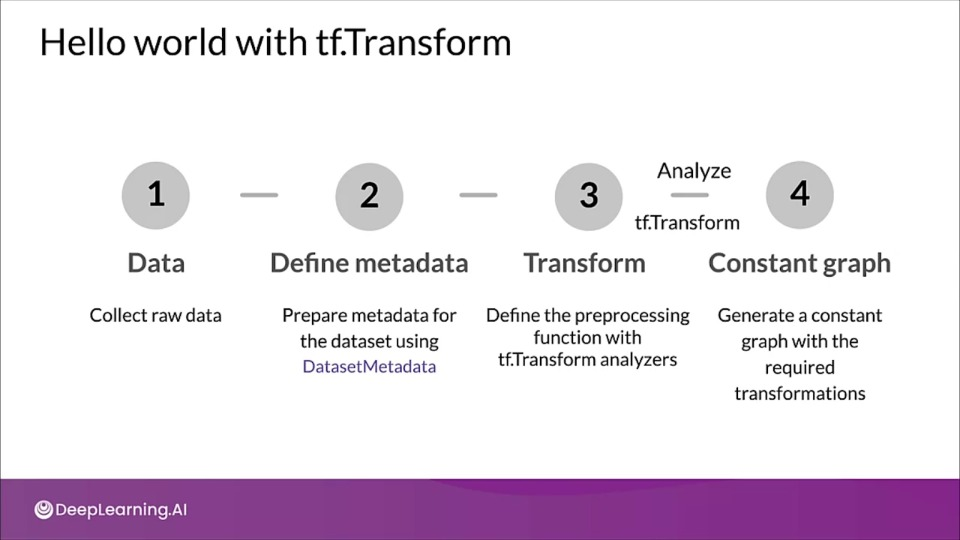### Inspect data and prepare metadata

from tensorflow_transform.tf_metadata import (

# define sample data
raw_data = [
{'x': 1, 'y': 1, 's': 'hello'},
{'x': 2, 'y': 2, 's': 'world'},
{'x': 3, 'y': 3, 's': 'hello'}
]

dataset_schema.from_feature_spec({
'y': tf.io.FixedLenFeature([], tf.float32),
'x': tf.io.FixedLenFeature([], tf.float32),
's': tf.io.FixedLenFeature([], tf.string)
}))


### Preprocessing data (Transform)

def preprocessing_fn(inputs):
"""Preproceess input columns into transformed columns"""
x, y, s = inputs['x'], inputs['y'], inputs['s']
x_centered = x - tft.mean(x)
y_normalized = tft.scale_to_0_1(y)
s_integerized = tft.compute_and_apply_vocabulary(s)
# feature cross
x_centered_times_y_normalized  (x_centered * y_normalized)
return {
'x_centered': x_centered,
'y_normalized': y_normalized,
's_integerized': s_integerized,
'x_centered_times_y_normalized': x_centered_times_y_normalized,
}


### Running the pipeline

def main():
with tft_beam.Context(temp_dir=tempfile.mkdtemp()):
# Define a beam pipeline
transformed_dataset, transform_fn = (
preprocessing_fn))

print('\nRaw data:\n{}\n'.format(pprint.pformat(raw_data)))
print('\Transformed data:\n{}'.format(pprint.pformat(tranformed_Data)))

if __name__ == '__main__':
main()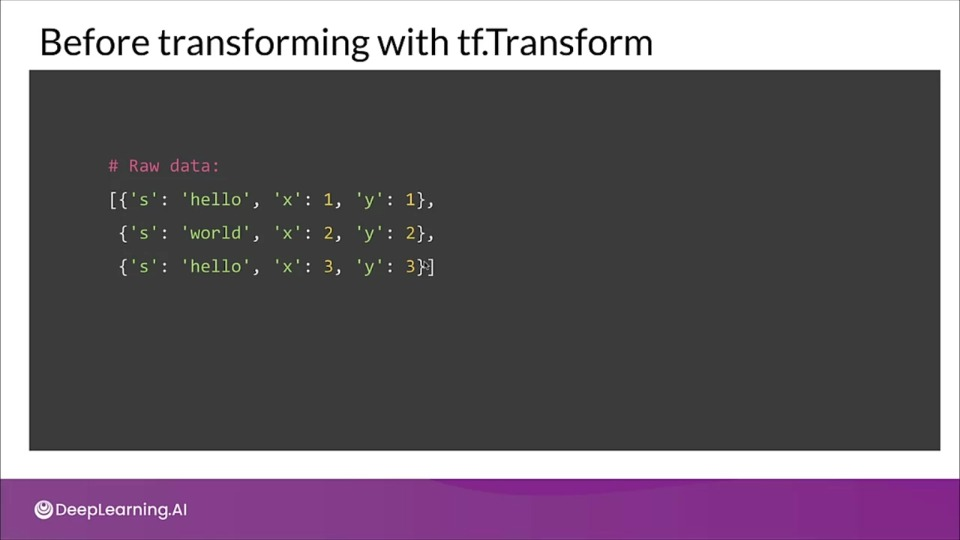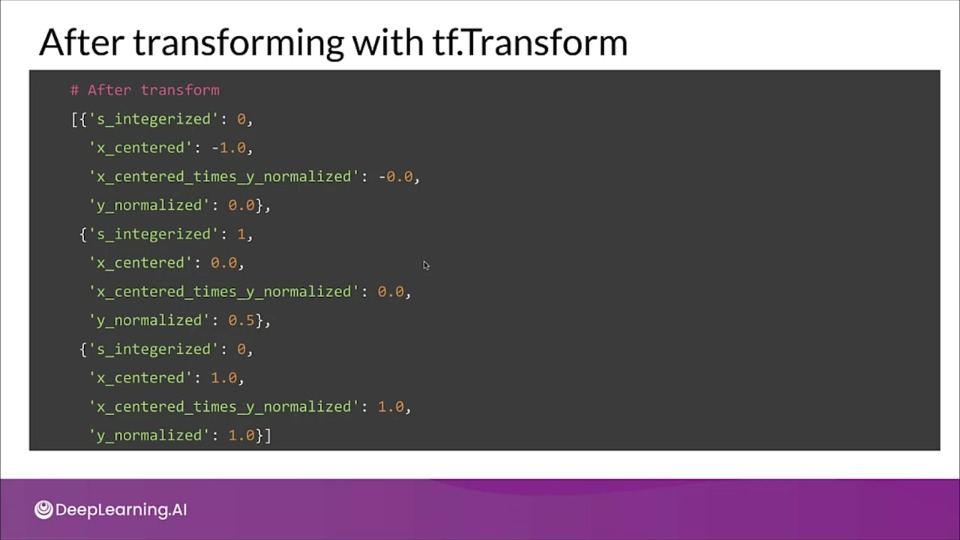### Key points

• tf.Transform allows the preprocessing of input data and creating features
• tf.Transform allows defining pre-processing pipelines and their execution using large-scale data processing frameworks, like Apache Beam.
• In a TFX pipeline, the Transform component implements feature engineering using TensorFlow Transform

## Feature Selection — Feature Spaces

• N dimensional space defined by your N features
• Not including the target label

### Feature space coverage

• Train/Eval datasets should be representative of the serving dataset
• Same numerical ranges
• Same classes
• Similar characteristics for image data
• Similar vocabulary.

### Ensure feature space coverage

• Data affected by: seasonality, trend, drift
• Serving data: new values in features and labels
• Continuous monitoring, key for success!

## Feature Selection

• Feature selection identifies the features that best represent the relationship between the features, and the target that we're trying to predict.
• Remove features that don't influence the outcome
• Reduce the size of the feature space
• Reduces the resource requirements and model complexity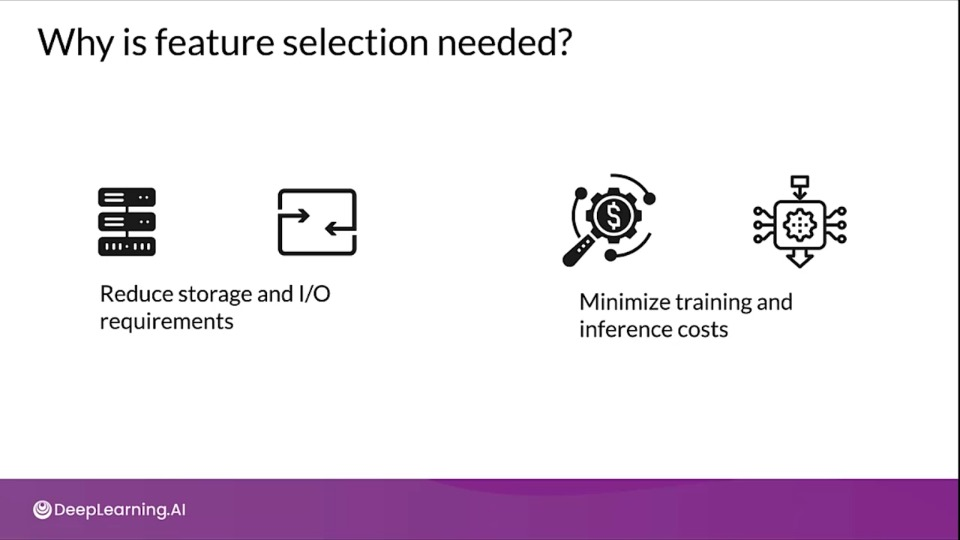### Unsupervised Feature selection methods

• Feature-target variable relationship not considered
• Removes redundant features (correlation)
• Two features that are highly correlated, you might need only one

### Supervised feature selection

• Uses features-target variable relationship
• Selects those contributing the most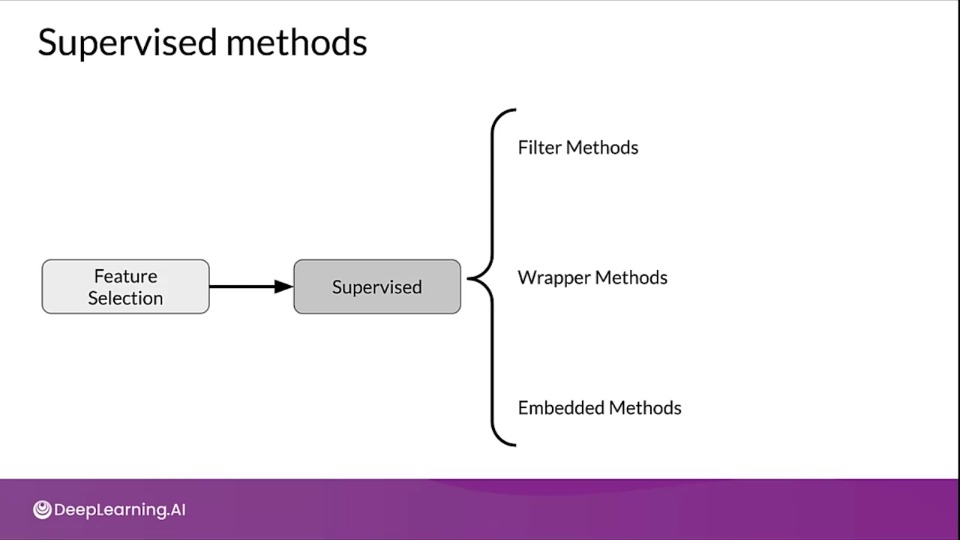## Filter methods

• Correlated features are usually redunfant
• We remove them.
• Filter methods suffer from inefficiencies as they need to look at all the possible feature subsets

Popular filter methods:

• Pearson Correlation
• Between features, and between the features and the label
• Univariate Feature Selection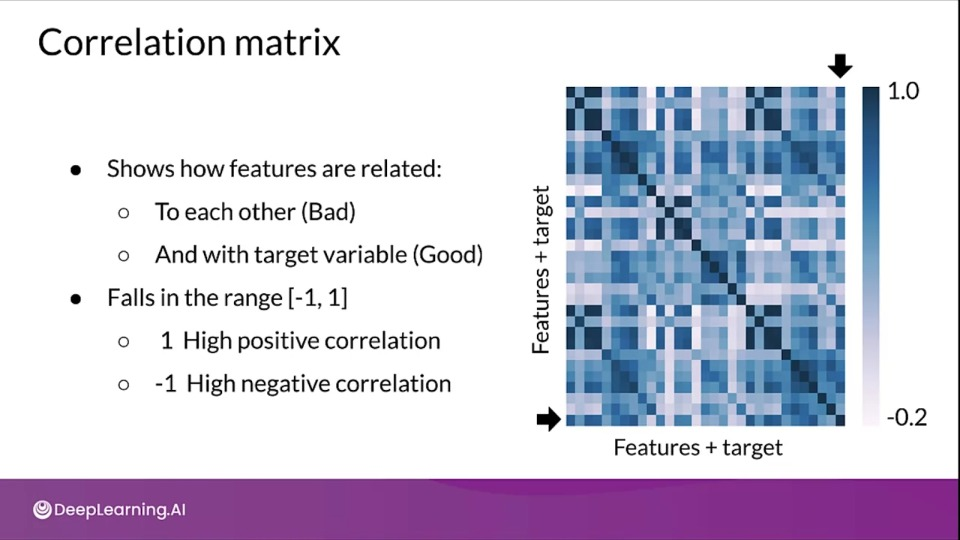### Feature comparison statistical tests

• Pearson's correlation: Linear relationships
• Kendall Tau Rank Correlation Coefficient: Monotonic relationships & small sample size
• Spearman's Rank Correlation Coefficient: Monotonic relationships

Other methods:

• Pearson Correlation (numeric features - numeric target, exception: when target is 0/1 coded)
• ANOVA f-test (numeric features - categorical target)
• Chi-squared (categorical features - categorical target)
• Mutual information

### Determining correlation

# Pearson's correlation by default
cor = df.corr()

plt.figure(figsize=(20,20))
import seaborn as sns
sns.heatmap(cor, annot=True, cmap=plt.cm.PuBu)
plt.show()


### Selecting Features

cor_target = abs(cor['diagnosis_int'])

# Selecting highly correlated features as potential features to eliminate
relavant_features = cor_target[cor_target > 0.2]


### Univariate feature selection in Sklearn

Sklearn Univarite feature selection routines:

1. SelectKBest
2. SelectPercentile
3. GenericUnivariateSelect

Statistical tests available:

• Regression: f__regression, mutual_info_regression
• Classification: chi2 , f_classif, mutual_info_classif

### SelectKBest implementation

def univariate_selection():
X_train, X_test, y_train, y_test = train_test_split(X, y,
test_size=0.2, stratify=y, random_state=123)
X_train_scaled = StandardScaler().fit_transform(X_train)
X_test_scaled = StandardScaler().fit(X_train).transform(X_test)

min_max_scaler = MinMaxScaler()
scaled_X = min_max_scaler.fit_transform(X_train_scaled)

selector = SelectKBest(chi2, k=20) # Use Chi-Squared test
X_new = selector.fit_transform(scaled_X, y_train)
feature_idx = selector.get_support()
feature_names = df.drop("diagnosis_int", axis=1).columns[feature_idx]
return feature_names


## Wrapper Methods

It's a search method against the features that you have using a model as the measure of their effectiveness

Wrapper methods are based on greedy algorithm and this solutions are slow to compute.

Popular methods include:

1. Forward Selection
2. Backward Elimiation
3. Recursive Feature Elimination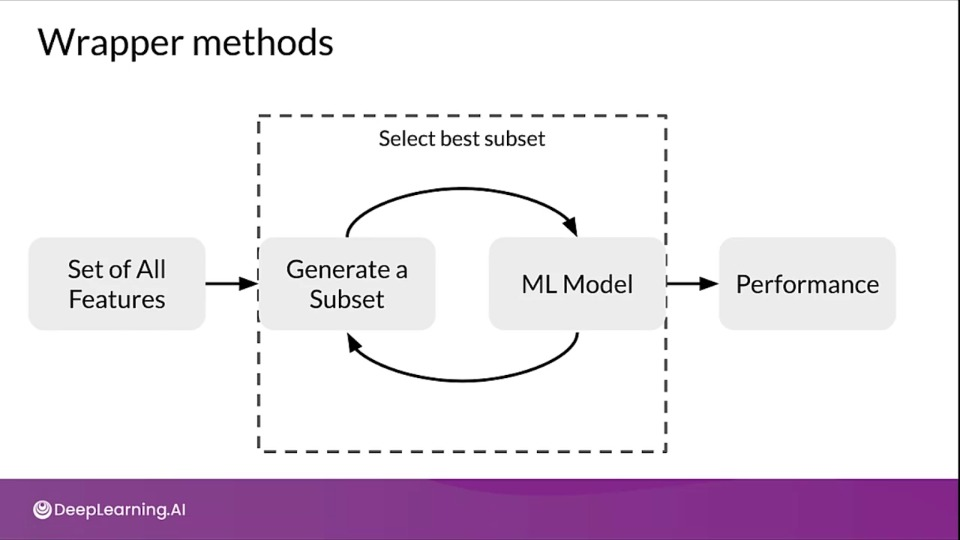### Forward Selection

1. Iterative, greedy method
2. Starts with 1 feature
3. Evaluate model performance when adding each of the additional features, one at a time.
4. Add next feature that gives the best performance
5. Repeat until there is no improvement

### Backward Elimination

2. Evaluate model performance when removing each of the included features, one at a time.
3. Remove next feature that gives the best performance
4. Repeat until there is no improvement

### Recursive Feature Elimination (RFE)

1. Select a model to use for evaluating feature importance
2. Select the desired number of features
3. Fit the model
4. Rank features by importance
6. Repeat until the desired number of features remains
def run_rfe():
X_train, X_test, y_train, y_test = train_test_split(X, y,
test_size=0.2, stratify=y, random_state=123)

X_train_scaled = StandardScaler().fit_transform(X_train)
X_test_scaled = StandardScaler().fit(X_train).transform(X_test)

model = RandomForestClassifier(criterion='entropy', random_state=47)
rfe = RFE(model, 20)
rfe = rfe.fit(X_train_scaled, y_train)
feature_names = df.drop('diagnosis_int', axis=1).columns[rfe.get_support()]
return feature_names

rfe_feature_names = run_rfe()

rfe_eval_df = evaluate_model_on_features(df[rfe_feature_names], y)


## Embedded Methods

• L1 regularization
• Feature importance

### Feature importance

• Assigns scores for each feature in data
• Discard features scores lower by feature importance

### Feature importance with Sklearn

• Feature Importance class is in-built in Tree Based Model (e.g., RandomForestClassifier)
• Feature importance is available as a property feature_importances_
• We can then use SelectFromModel to select features from the trained model based on assigned feature importances.
def feature_importances_from_tree_based_model_():
X_train, X_test, y_train, y_test = train_test_split(X, y,
test_size=0.2, stratify=y, random_state=123)

model = RandomForestClassifier()
model = model.fitX_Train y_train)

feat_importances = pd.Series(model.feature_importances_, index=X.columns)
feat_importances.nlargest(10).plot(kind='barh')
plt.show()
return model


### Select features based on importance

def select_features_from_model(model):
model = SelectFromModel(model, prefit=True, threshold=0.012)
feature_idx = df.drop("diagnosis_int", 1).columns[feature_idx]
return feature_names


### Tying together and evaluation

# Calcualte and plot feature importances
model = feature_importances_from_tree_based_model_()

# Select fearues based on feature importances
feature_imp_feature_names = select_features_from_model(model)

Week 2 References

## Week 2: Feature Engineering, Transformation and Selection

If you wish to dive more deeply into the topics covered this week, feel free to check out these optional references. You won’t have to read these to complete this week’s practice quizzes.

Mapping raw data into feature

Feature engineering techniques

Scaling

Facets

Embedding projector

Encoding features

TFX: